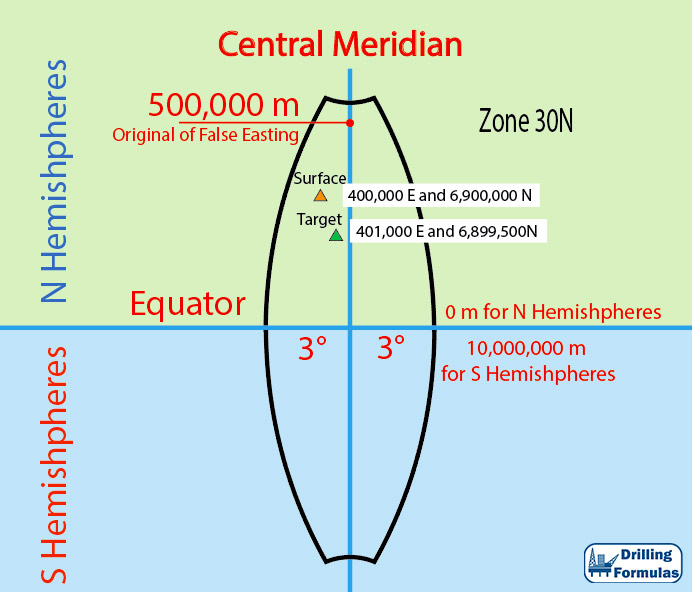# Scale Factor in UTM Mapping System and its Application in Directional Drilling

Scale factor represents distortion from a mapping system since the Earth is mapped into a flat surface, but the actual surface is in curvature. Figure 1 illustrates a scale factor with a reference location of the Earth’s surface. If the location is above the map projection plan, the scale factor will be less than 1.0. However, if the location is below the mapping projection, the scale factor will be more than 1.0. A scale factor less than 1 means that the actual distance on the Earth’s surface is longer than the actual distance on the map. Whereas, the scale factor of more than 1 demonstrates that the actual distance on the Earth is shorter than the map distance.Figure 1 – Scale Factor

For UTM, the central meridian (CM) has a scale factor of 0.9996. The locations where the scale factor is one are 320,000m E and 680,000 E (Figure 2).Figure 2 – Scale Factor Depending on Location for each UTM zone

Example:

This example demonstrates how to use the scale factor to calculate the distance projected in the map.

The surface location is located in UTM 30N. The location coordination is 400,000 E and 6,900,000 N. and the target is located at 401,000 E and 6,899,500N and the UTM zone is 30N. The scale factor at the location is 0.999685. The surface and the target for this  can be illustrated in Figure 3.Figure 3- Surface and Target Location

Distance from Surface Location to Target

ΔN = 6,899,500 – 6,900,000 = – 500 m

ΔE = 401,000 – 400,000 = 1,000 mMap Distance = 1118.034 mFigure 4 – Distance from surface to the target illustration

True Distance

This distance must be applied to the scale factor in order to get the true distance.

True Distance (m) = Map Distance ÷ scale factor

True Distance (m) = 1118.034 ÷ 0.999685 = 1118.3863 m

The true distance is the horizontal displacement referencing from the surface location to the target.

Target Azimuth

The target in reference to the surface location is located in 2nd quadrant; therefore the target azimuth is calculated by the following relationship;

Target AZI = 90 + tan-1(ΔN÷ΔE)

Target AZI = 90 + tan-1((500) ÷ (1000))

Target AZI = 116.565 degree

References

Adam Bourgoyne, 2014. Directional Drilling and Deviation Control: Definitions and Reasons for Directional Drilling. 1st Edition.

French Oil & Gas Industry Association, 1990. Directional Drilling and Deviation Control. Edition. Technip Editions.

Tom Inglis, 1988. Directional Drilling (Petroleum Engineering and Development Studies) (v. 2). 1987 Edition. Springer.

### Related Post

Shape of the Earth and Geodetic Datums for Directi... Directional drilling relies on mapping system to accurately identify location of the wells. Therefore, it is very important to learn the basics of map...
Directional Drilling Disaster ? Have you ever seen this VDO? In the VDO, it stated that this was a false calculation which was result this directional drilling disaster. However, ...
Why Directional Wells Are Drilled? The directional drilling, whereby a bit/BHA is precisely guided through a particular target, is a fascinating one. This article will describe about ap...
Latitude, Longitude and Drilling Map Projection Directional drilling maps are flat, but the Earth is an oblate spheroid. This leads to a challenge to accurately represent the wellbore position on th...
Share the joy
• 514
•
•
•
•
•
•
•
•
514
Shares